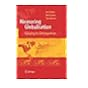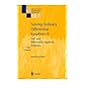Normal view

# Tensor Spaces and Numerical Tensor Calculus [ Livre] / Wolfgang, Hackbusch

Langue: Anglais ; de l'oeuvre originale, Anglais.Mention d'édition: Second editionPublication : Springer Nature Switzerland AG, 2019Description : XXVIII, 605 pagesISBN: 9783030355531.Classification: 518 Analyse numérique, modélisation et calcul scientifiqueRésumé: Special numerical techniques are already needed to deal with n × n matrices for large n. Tensor data are of size n × n ×...× n=nd, where nd exceeds the computer memory by far. They appear for problems of high spatial dimensions. Since standard methods fail, a particular tensor calculus is needed to treat such problems. This monograph describes the methods by which tensors can be practically treated and shows how numerical operations can be performed. Applications include problems from quantum chemistry, approximation of multivariate functions, solution of partial differential equations, for example with stochastic coefficients, and more. In addition to containing corrections of the unavoidable misprints, this revised second edition includes new parts ranging from single additional statements to new subchapters. The book is mainly addressed to numerical mathematicians and researchers working with high-dimensional data. It also touches problems related to Geometric Algebra..Sujet - Nom commun: Analyse numérique
Current location Call number Status Notes Date due Barcode
ENS Rennes - Bibliothèque
Mathématiques
518 HAC (Browse shelf) Available 518 Analyse numérique, modélisation et calcul scientifique 043871
##### Browsing ENS Rennes - Bibliothèque shelves, Shelving location: Mathématiques Close shelf browser518 GOD Numerical approximation of hyperbolic systems of conservation laws 518 GRE Iterative methods for solving linear systems 518 GUE Méthodes d'approximation, équations différentielles, applications Scilab 518 HAC Tensor Spaces and Numerical Tensor Calculus 518 HAI Solving ordinary differential equations. I, Nonstiff problems 518 HAI Solving ordinary differential equations 518 HER Analyse numérique

Special numerical techniques are already needed to deal with n × n matrices for large n. Tensor data are of size n × n ×...× n=nd, where nd exceeds the computer memory by far. They appear for problems of high spatial dimensions. Since standard methods fail, a particular tensor calculus is needed to treat such problems. This monograph describes the methods by which tensors can be practically treated and shows how numerical operations can be performed. Applications include problems from quantum chemistry, approximation of multivariate functions, solution of partial differential equations, for example with stochastic coefficients, and more. In addition to containing corrections of the unavoidable misprints, this revised second edition includes new parts ranging from single additional statements to new subchapters. The book is mainly addressed to numerical mathematicians and researchers working with high-dimensional data. It also touches problems related to Geometric Algebra.